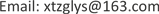1. 文章中用到的常数

2. 普朗克尺度、普朗克黑洞

L P = 2 G m P / c 2 (1)

mp——普朗克黑洞的质量。

Δ r Δ p = h / 2 π

L P m P c = h / 2 π (2)

(1) × (2)得： L P 2 = 2 G h / 2 π c 3 = G h / π c 3

L P = ( G h / π c 3 ) 1 / 2

3. 黑洞的熵及黑洞的辐射温度与辐射3.1. 黑洞熵公式的推导

S = n A (n为比例常数)

S P = k log w P = n A P (3)

(SP、wP、AP分别为普朗克黑洞的熵、微观状态数和表面积)。

(3)中对数的底数取wP时， log w P = 1 , k = n A P , n = k / A P

S = n A = A k c 3 / 4 G h (4)

S = 4 π k G m 2 / c h (m为黑洞的质量) (5)

( S = k B ln w )玻尔兹曼常数kB之关系。

k = m P c 2 / T P (6)

P V = ( N R / N 0 ) T

P V = N k B T (7)

P P V P = N k B T P

P P V P = k B T P

Q = m P c 2 + P P V P

m P c 2 − P P V P = 0 ； P P V P = m P c 2

3.2. 黑洞的辐射温度

T B = d Q / d S = d ( m c 2 ) / d S = c 2 d m / d S

T B = c 2 / ( d S / d m )

T B = c 2 / ( 8 π k G m / c h )

T B = c 3 h / 8 π k G m (9)

T P = m P c 2 / k B

3.3. 黑洞的辐射(霍金辐射)

4. 宇宙空间暴胀机制

V r − 1 T = 衡 量

T S = ( L P / R P ) 2 T P

T B = ( G h / 2 π c 3 L P R B ) T P

T S = ( 1 / a b ) 2 T P , T B = ( 1 / 2 b ) T P

2) 当 b = 2 时， a = 1 ， R S = R B = 2 L P 空间半径与黑洞半径相等；

3) 当 b > 2 时， a < 1 ， R S < R B 空间半径小于黑洞半径。

1) 表示空间与黑洞等温同步膨胀，空间半径始终大于黑洞半径；

2) 表示空间与黑洞同步膨胀至半径均等于二倍的普朗克尺度，此时的温度为： T B = T P / 4 ；

3) 表示如果继续膨胀，黑洞的半径将比空间的半径要大，温度也要比空间的温度高。然而这种情况是不可能发生的，此刻黑洞将会以霍金辐射的方式剧烈蒸发，从而致使空间以爆发式增长，即发生暴胀。

T S = ( L P / R S ) 2 T P = T P / a 2 (12)

T B = ( L P / 2 R B ) T P = T P / 2 a (13)

(12)/(13)得： T S / T B = 2 / a 。

2) 当 a = 2 ， R S = R B = 2 L P 时， T S = T B ，空间温度与黑洞温度相等；

3) 当 a > 2 ， R S = R B > 2 L P 时， T S < T B ，空间温度低于黑洞温度。

(1)、(2)两种情况说明，空间与黑洞等径膨胀直至 R S = R B = 2 L P ；

(3)表明，如果继续膨胀，空间温度将低于黑洞温度，此刻黑洞将会以霍金辐射的方式剧烈蒸发，从而使空间的爆发式增长，以至发生暴胀。

5. 结论

1) 普朗克尺度 L P = ( G h / π c 3 ) 1 / 2 。

2) 黑洞的熵 S = A k c 3 / 4 G h = 4 π k G m 2 / c h ，其中的玻尔兹曼常数k就是自然对数条件下( S = k B ln w )的玻尔兹曼常数kB；黑洞的辐射温度 T B = c 3 h / 8 π k G m 。

3) 普朗克黑洞的熵等于自然对数条件下的玻尔兹曼常数，即 S P = k B ；普朗克黑洞的微观状态数等于自然数，即 w P = e 。

4) 黑洞可通过空间的量子涨落而产生辐射。

5) 宇宙原始空间与黑洞的相互作用使空间发生暴胀。